# CBSE Class 10 Mathematics Quadratic Equations Worksheet Set B

Download printable Mathematics Class 10 Worksheets in pdf format, CBSE Class 10 Mathematics Quadratic Equations Worksheet Set B has been prepared as per the latest syllabus and exam pattern issued by CBSE, NCERT and KVS. Also download free pdf Mathematics Class 10 Assignments and practice them daily to get better marks in tests and exams for Grade 10. Free chapter wise worksheets with answers have been designed by Standard 10 teachers as per latest examination pattern

## Worksheet for Class 10 Mathematics Chapter 4 Quadratic Equation

Class 10 Mathematics students should refer to the following printable worksheet in Pdf for Chapter 4 Quadratic Equation in Grade 10. This test paper with questions and solutions for Standard 10 Mathematics will be very useful for tests and exams and help you to score better marks

### Chapter 4 Quadratic Equation Class 10 Mathematics Worksheet Pdf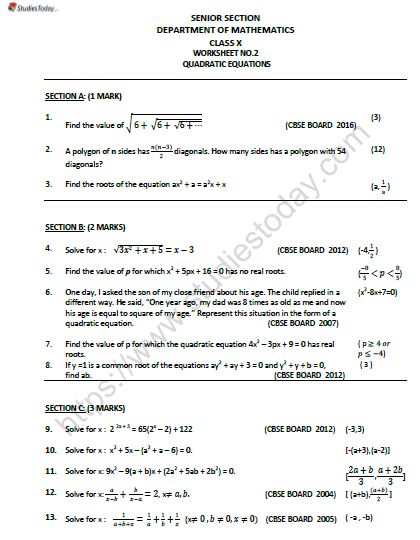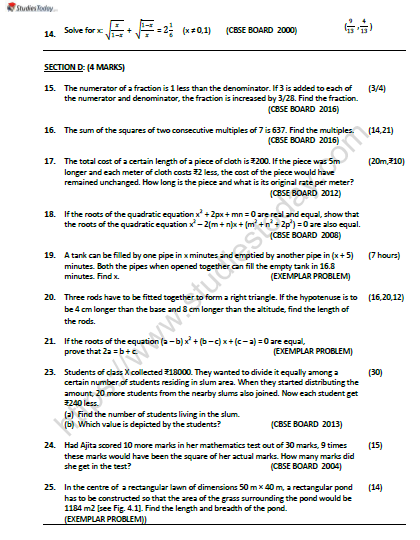VERY SHORT ANSWER TYPE QUESTIONS :

Question. If one of the roots of x2 + px − 4 = 0 is − 4 then find the product of its roots and the value of p.
Answer : If − 4 is a root of the quadratic equation,
x2 + px − 4 = 0
∴ (− 4)2 + (− 4) (p) − 4 = 0
⇒ 16 − 4p − 4 = 0
⇒ 12 − 4p = 0 ⇒ p = 3
Now, in ax2 + bx + c = 0, the product of the roots = c/a
∴ Product of the roots in x2 − px − 4 = 0
= − 4/1 = − 4

Question. For what value of k are the roots of the quadratic equation kx2 + 4x + 1 = 0 equal and real?
Answer : Comparing kx2 + 4x + 1 = 0, with ax2 + bx + c = 0, we get
a = k
b = 4
c = 1
∴ b2 − 4ac = (4)2 − 4 (k) (1)
= 16 − 4k
For equal and real roots, we have
b2 − 4ac = 0
⇒ 16 − 4k = 0
⇒ 4k = 16
⇒ k = 16/4 = 4

x2 − 2ax + (a2 − b2) = 0
Answer : Comparing x2 − 2ax + (a2 − b2) = 0, with ax2 + bx + c = 0, we have:
a = 1, b = − 2a, c = a2 − b2
∴ x = − b ± √b2 − 4ac/2a
⇒ x = − (− 2a) ± − √(2a)2 − 4 (1) (a2 − b2)/2)1_
= 2a ± √4a2 − 4a− 4b2
= 2a ± √4b2/2 = 2a ± b/2 = a ± b
∴ x = (a + b) or x = (a − b)

Question. For what value of k, does the quadratic equation x2 − kx + 4 = 0 have equal roots?
Answer : Comparing x2 − kx + 4 = 0 with ax2 + bx + c = 0, we get
a = 1
b = − k
c = 4
∴ b2 − 4ac = (− k)2 − 4 (1) (4) = k2 − 16
For equal roots,
b2 − 4ac = 0
⇒ k2 − 16 = 0
⇒ k2 = 16
⇒ k = ± 4

Question. If the roots of the quadratic equation − ax2 + bx + c = 0 are equal then show that b2 = 4ac.
Answer : ä For equal roots, we have
b2 − 4ac = 0
∴ b2= 4ac

Question. If one root of the quadratic equation 2x2 − 3x + p = 0 is 3, find the other root of the quadratic equation. Also find the value of p.
2x2− 3x + p = 0
∴ a = 2, b = − 3 and c = p
Since, the sum of the roots = − b/a
= − (−3)/2 = 3/2
∴ One of the roots = 3
∴ The other root = 3/2 − 3 = − 3/2
Now, substituting x = 3 in (1), we get
2 (3)2 − 3 (3) + p = 0
⇒ 18 − 9 + p = 0
⇒ 9 + p = 0 ⇒ p = − 9

Question. For what value of k does (k − 12) x2 + 2 (k − 12) x + 2 = 0 have equal roots?
Answer : Comparing (k − 12) x2 + 2 (k − 12) x + 2 = 0 with ax2 + bx + c = 0, we have:
a = (k − 12)
b = 2 (k − 12)
c = 2
∴ b2 − 4ac = [2 (k − 12)]2 − 4 (k − 12) (2)
= 4 (k − 12)2 − 8 (k − 12)
= 4 (k − 12) [k − 12 − 2]
= 4 (k − 12) (k − 14)
For equal roots,
b2 − 4ac = 0
⇒ 4 (k − 12) [k − 14] = 0
⇒ Either 4 (k − 12) = 0 ⇒ k = 12
or k − 14 = 0 ⇒ k = 14
But k = 12 makes k − 12 = 0 which is not required
∴ k ≠ 12
⇒ k = 14

Question. For what value of k, does the given equation have real and equal roots?
(k + 1) x2 − 2 (k − 1) x + 1 = 0.
Answer : Comparing the given equation with ax2 + bx + c = 0, we have:
a = k + 1
b = − 2 (k − 1)
c = 1
For equal roots, b2 − 4ac = 0
∴ [− 2 (k − 1)]2 − 4 (k + 1) (1) = 0
⇒ 4 (k − 1)2 − 4 (k + 1) = 0
⇒ 4 (k2 + 1 − 2k) − 4k − 4 = 0
⇒ 4k2 + 4 − 8k − 4k − 4 = 0
⇒ 4k2 − 12k = 0
⇒ 4k (k − 3) = 0
⇒ k = 0 or k = 3

Question. For what value of k does the equation 9x2 + 3kx + 4 = 0 has equal roots?
Answer : Comparing 9x2 + 3kx + 4 = 0 with ax2 + bx + c = 0, we get
a = 9
b = 3k
c = 4
∴ b2 − 4ac = (3k)2 − 4 (9) (4)
= 9k2 − 144
For equal roots,
b2 − 4ac = 0
⇒ 9k2 − 144 = 0
⇒ 9k2 = 144
⇒ k2 = 144/9 = 16
⇒ k = ± 4

Question. What is the nature of roots of the quadratic equation 4x2 − 12x + 9 = 0?
Answer : Comparing 4x2 − 12x + 9 = 0 with ax2 + bx + c = 0 we get
a = 4
b = − 12
c = 9
∴ b2 − 4ac = (− 12)2 − 4 (4) (9)
= 144 − 144 = 0
Since b2 − 4ac = 0
∴ The roots are real and equal.

Question. If one of the roots of the quadratic equation 2x2 + kx − 6 = 0 is 2, find the value of k. Also find the other root.
2x2 + kx − 6 = 0
one root = 2
Substituting x = 2 in 2x2 + kx − 6 = 0
We have:
2 (2)2 + k (2) − 6 = 0
⇒ 8 + 2k − 6 = 0
⇒ 2k + 2 = 0 ⇒ k = − 1
∴ 2x2 + kx − 6 = 0 ⇒ 2x2 − x − 6 = 0
Sum of the roots = − b/a = 1/2
∴ other root = 1/2 − 2
= 3/2

Question. Find the value of ‘k’ for which the quadratic equation kx2 − 5x + k = 0 have real roots.
Answer : Comparing kx2 − 5x + k = 0 with ax2+ bx + c = 0, we have:
a = k
b = − 5
c = k
∴ b2 − 4ac = (− 5)2 − 4 (k) (k)
= 25 − 4k2
For equal roots, b2 − 4ac = 0
∴ 25 − 4k2 = 0
⇒ 4k2 = 25
⇒ k2 = 25/4
⇒ k = ±√25/4 = ±5/2

Question. Write the value of k for which the quadratic equation x2 − kx + 9 = 0 has equal roots.
Answer : Comparing x2 − kx + 9 = 0 with ax2 + bx + c = 0, we get
a = 1
b = − k
c = 9
∴ b2 − 4ac = (− k)2 − 4 (1) (9)
= k2 − 36
For equal roots,
b2 − 4ac = 0
⇒ k2 − 36 = 0 ⇒ k2 = 36
⇒ k = ± 6

Question. If − 4 is a root of the quadratic equation x2 + px − 4 = 0 and x2 + px + k = 0 has equal roots, find the value of k.
Answer : ∴ (–4) is a root of x2 + px − 4 = 0
∴ (− 4)2 + p (− 4) = 0
⇒ 16 − 4p − 4 = 0
⇒ 4p = 12 or p = 3
Now, x2+ px + k = 0
⇒ x2 + 3x + k = 0 [ ∴ p = 3]
Now, a = 1, b = 3 and c = + k
∴ b2 − 4ac = (3)2 − 4 (1) (k)
= 9 − 4k
For equal roots, b2 − 4ac = 0
⇒ 9 − 4k = 0 ⇒ 4k = 9
⇒ k = 9/4

Question. Determine the value of k for which the quadratic equation 4x2 − 4kx + 1 = 0 has equal roots.
4x2 − 4kx + 1 = 0
Comparing with ax2 + bx + c = 0, we have
a = 4, b = − 4k and c = 1
∴ b2 − 4ac = (− 4k)2 − 4 (4k) (1)
= 16k2 − 16
For equal roots
b2 − 4ac = 0
∴ 16k2 − 16 = 0
⇒ 16k2 = 16 ⇒ k2 = 1
⇒ k = ± 1

Question. For what value of k are the roots of the quadratic equation 3x2 + 2kx + 27 = 0 real and equal?
Answer : Comparing 3x2 + 2 kx + 27 = 0 with ax2+ bx + c = 0, we have:
a = 3
b = 2k
c = 27
∴ b2 − 4ac = (2k)2 − 4 (3) (27)
= 4k2 − (12 × 27)
For the roots to be real and equal
b2 − 4ac = 0
⇒ 4k2 − (12 × 17) = 0
⇒ 4k= 12 × 27
⇒ k2 = 12 × 27/4 = 81
⇒ k = ± 9

Question. Solve for x: 36x2 − 12ax + (a2 − b2) = 0.
36x2 − 12ax + (a2 − b2) = 0
Comparing (1) with Ax2 + Bx + C = 0, we have:
A = 36
B = − 12a
C = (a2 − b2)
∴ B2 − 4AC = [− 12a]2 − 4 (36) [a2 − b2]
= 144 a2 − 144 (a2 − b2)
= 144 a2 − 144 a2 + 144 b2
= 144 b2
Since, x = − B ± √B2 − 4AC/2A
∴ x = − (− 12a) ± √144 b2/2 (36)
⇒ x = 12a ± 12b/72
Taking +ve sign, we have:
x = 12a + 12b/72
⇒ x = 12/72 (a + b) = 1/6 (a + b)
Taking −ve sign, we get
x = 12a − 12b/72 (a − b) = 1/6 (a − b)
Thus, the required roots are:
x = 1/6 (a + b) and x = 1/6 (a − b)

Question. Find the roots of the quadratic equation 2x2 - 5x - 2 = 0, using the quadratic formula.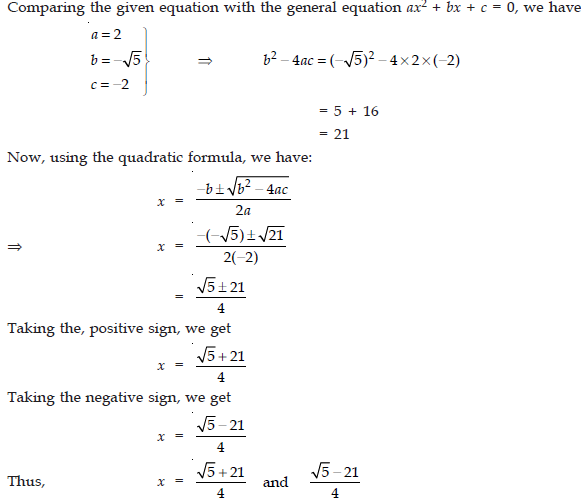Question. Find the roots of the equation:
1/x − 2 + 1/x = 8/2x + 5 : x ≠ 0, 2 −5/2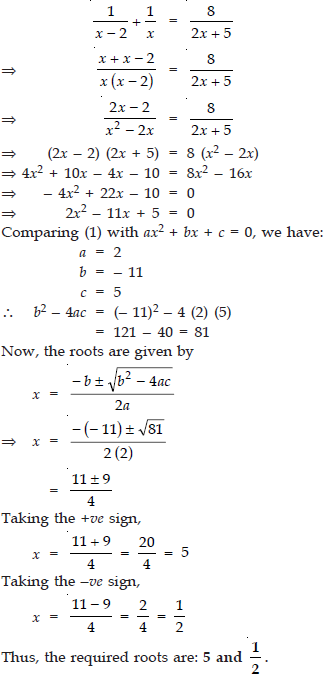Question. Solve 2x2 − 5x + 3 = 0.
2x2 − 5x + 3 = 0
Comparing (1) with ax2 + bx + c = 0,
∴ a = 2
b = − 5
c = 3
∴ b2 − 4ac = (− 5)2 − 4 (2) (3)
= 25 − 24 = 1
Since, x = − b ± √b2 − 4ac/2a
∴ x = − (− 5) ± √1/2 (2)
= 5 ± 1/4
Taking, + ve sign,
x = 5 + 1/4 = 6/4 = 3/2
Taking, −ve sign,
x = 5 − 1/4 = 4/4 = 1
Thus, the required roots are
x = 3/2 and x = 1

Question. Solve for x: 9x2 − 6ax + a2 − b2 = 0.
9x2 − 6ax + (a2 − b2) = 0
Comparing (1) with ax2 + bx + c = 0, we get
a = 9, b = − 6a and c = (a2 − b2)
∴ b2 − 4ac = (− 6a)2 − 4 (9) (a2 − b2)
= 36a2  − 36 (a2  − b2)
= 36a2  − 36a2  + 36b2
= 36b2 = (6b)2
Since, x = − b ± √b2 − 4ac/2a
∴ x = − (− 6a) ± 6√(6b)2/2(9)
⇒ x = 6a ± 6b/18
⇒ x = 6[a ± b]/18 = a ± b/3
a ± b a b
Taking the +ve sign, we get
x = a + b/3
Taking the −ve sign, we get
x = a − b/3
∴ The required roots are:
x = a + b/3 and x = a − b/3

Question. Evaluate √20 + √20 + √20 +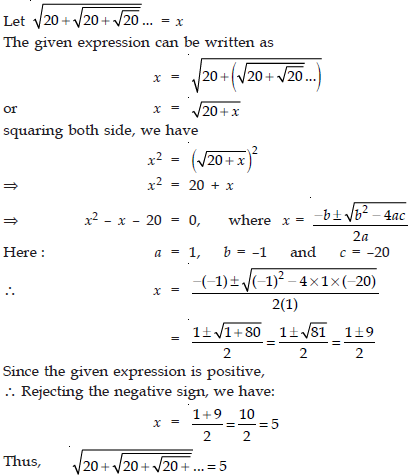Question. Find the roots of the equation:
1/x − 1/x − 3 = 4/3  ; x ≠ 0, 3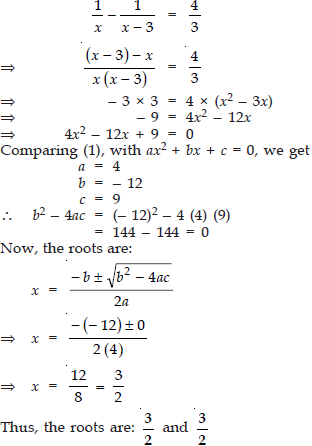Question. Solve the following quadratic equation:
2x2 + 4x − 8 = 0
2x2 + 4x − 8 = 0
Dividing by 2, we get
x2+ 2x − 4 = 0
Comparing (1) with ax2 + bx + c = 0,
a = 1,
b = 2
c = − 4
∴ b2 − 4ac = (2)2 − 4 (1) (− 4)
= 4 + 16 = 20
Since, x = − b ± √b2 − 4ac/2a
∴ x =
− 2 ± √20/2 (1)
⇒ x = − 2 ± 2√5/2
⇒ x = 2 [−1 ± √5]/2  = − 1 ± √5
Taking + ve sign, we get
x = [− 1 + √5]
Taking − ve sign, we get
x = [− 1 − √5]
Thus, the required roots are x = [− 1 + √5] and x = [− 1 − √5]

x2 − 4ax + 4a2 − b2 = 0
Answer : Comparing the given equation with ax2 + bx + c = 0, we have:
a = 1
b = − 4a
c = 4a2 − b2
∴ b2 − 4ac = [− (4a)]2 − 4 (1) [4a2 − b2]
= 16a2 − 4 (4a2 − b2)
= 16a2 − 16a2 + 4b2
= 4b2 = (2b)2
Since, x = − b ± √b2 − 4ac/2a
∴ x = − (− 4a) ± √(2b)2/2 (1)
⇒ x = 4a ± 2b/2
⇒ x = 2/2 [2a ± b] = 2a ± b
Taking the +ve sign, x = 2a + b
Taking the −ve sign, x = 2a − b
Thus, the required roots are:
x = 2a + b and x = 2a − b

x2 − 2ax + (a2 − b2) = 0
Answer : Comparing the given equation with ax2 + bx + c = 0, we have: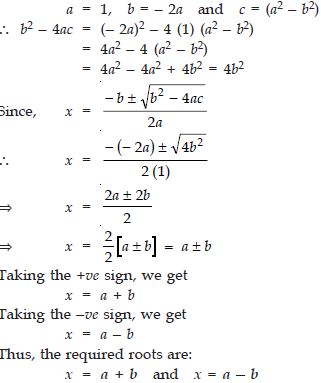Question. Solve: 16x2 − 8a2 x + (a4 − b4) = 0 for x.
16x2 − 8a2 x + a4 − b4 = 0
Comparing (1) with ax2 + bx + c = 0, we get
a = 16
b = − 8a2
c = (a4 − b4)
∴ b2 − 4ac = [− 8a2]2 − 4 (16) (a4 − b4)
= 64 a4 − 64 (a4 − b4)
= 64 a4 − 64 a4 + 64 b4
= 64 b4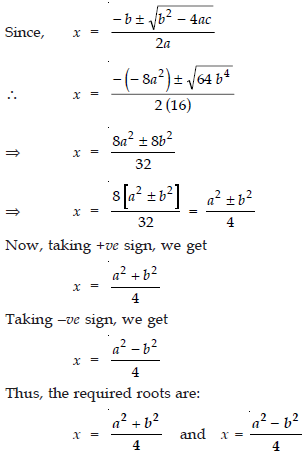x2 + 5x + 5 = 0
x2 + 5x + 5 = 0
Comparing (1) with ax2 + bx + c = 0, we have:
a = 1
b = 5
c = 5
∴ b2 − 4ac = (5)2 − 4 (1) (5)
= 25 − 20 = 5
Since, x = − b ± √b2 − 4ac/2a
∴ x = − 5 ± √5/2 (1)
⇒ x = − 5 ± √5/2
Taking +ve sign, we have:
x = − 5 + √5/2
Taking −ve sign, we have:
x = − 5 − √5/2
Thus, the required roots are:
x = − 5 + √5/2 and x = − 5 − √5/2

Question. Find the roots of the following equation:
1/x + 4 − 1/x − 7 = 11/30; x = 4, 7
1/x + 4 − 1/x − 7 = 11/30Extra Based Questions :

Check whether the following are quadratic equations:

Question. (x + 1)2 = 2(x − 3)
Answer : (x + 1)2 = 2(x − 3)
We have:
(x + 1)2 = 2 (x − 3)
⇒ x2 + 2x + 1 = 2x − 6
⇒ x2 + 2x + 1 − 2x + 6 = 0
⇒ x2 + 7 = 0
Since x2 + 7 is a quadratic polynomial
∴ (x + 1)2 = 2(x − 3) is a quadratic equation.

Question. x2 − 2x = (−2) (3 − x)
Answer : x2 − 2x = (− 2) (3 − x)
We have:
x2 − 2x = (− 2) (3 − x)
⇒ x2 − 2x = − 6 + 2x
⇒ x2 − 2x − 2x + 6 = 0
⇒ x2 − 4x + 6 = 0
Since x2 − 4x + 6 is a quadratic polynomial
∴ x2 − 2x = (−2) (3 − x) is a quadratic equation.

Question. (x − 2) (x + 1) = (x − 1) (x + 3)
Answer : (x − 2) (x + 1) = (x − 1) (x + 3)
We have:
(x − 2) (x + 1) = (x − 1) (x + 3)
⇒ x2 − x − 2 = x2 + 2x − 3
⇒ x2 − x − 2 − x2 − 2x + 3 = 0
⇒ −3x + 1 = 0
Since −3x + 1 is a linear polynomial
∴ (x − 2) (x + 1) = (x − 1) (x + 3) is not quadratic equation.

Question. (x − 3) (2x + 1) = x(x + 5)
Answer : (x − 3) (2x + 1) = x(x + 5)
We have:
(x − 3) (2x + 1) = x (x + 5)
⇒ 2x2 + x − 6x − 3 = x2 + 5x
⇒ 2x2 − 5x − 3 − x2 − 5x = 0
⇒ x2 + 10x − 3 = 0
Since x2 + 10x − 3 is a quadratic polynomial
∴ (x − 3) (2x + 1) = x(x + 5) is a quadratic equation.

Question. (2x − 1) (x − 3) = (x + 5) (x − 1)
Answer : (2x − 1) (x − 3) = (x + 5) (x − 1)
We have:
(2x − 1) (x − 3) = (x + 5) (x − 1)
⇒ 2x2 − 6x − x + 3 = x2 − x + 5x − 5
⇒ 2x2 − x2 − 6x − x + x − 5x + 3 + 5 = 0
⇒ x2 − 11x + 8 = 0
Since x2 − 11x + 8 is a quadratic polynomial
∴ (2x − 1) (x − 3) = (x + 5) (x − 1) is a quadratic equation.

Question. x2 + 3x + 1 = (x − 2)2
Answer : x2 + 3x + 1 = (x − 2)2
We have:
x2+ 3x + 1 = (x − 2)2
⇒ x2 + 3x + 1 = x2 − 4x + 4
⇒ x2 + 3x + 1 − x2 + 4x − 4 = 0
⇒ 7x − 3 = 0
Since 7x − 3 is a linear polynomial.
∴ x2 + 3x + 1 = (x − 2)2 is not a quadratic equation.

Question. (x + 2)3 = 2x(x2 − 1)
Answer : (x + 2)3 = 2x(x2− 1)
We have:
(x + 2)3 = 2x(x2 − 1)
⇒ x3 + 3x2(2) + 3x(2)2 + (2)3 = 2x3 − 2x
⇒ x3 + 6x2 + 12x + 8 = 2x3 − 2x
⇒ x3 + 6x2 + 12x + 8 − 2x3 + 2x = 0
⇒ − x3 + 6x2 + 14x + 8 = 0
Since −x3 + 6x2 + 14x + 8 is a polynomial of degree 3
∴ (x + 2)3 = 2x(x2 − 1) is not a quadratic equation.

Question. x3 − 4x2 − x + 1 = (x − 2)3
Answer : x3 − 4x2 − x + 1 = (x − 2)3
We have:
x3 − 4x2 − x + 1 = (x − 2)3
⇒ x3 − 4x2 − x + 1 = x3 + 3x2(− 2) + 3x(− 2)2 + (− 2)3
⇒ x3 − 4x2 − x + 1 = x3 − 6x2 + 12x − 8
⇒ x3− 4x2 − x − 1 − x3 + 6x2 − 12x + 8 = 0
⇒ 2x2 − 13x + 9 = 0
Since 2x2 − 13x + 9 is a quadratic polynomial
∴ x3 − 4x2 − x + 1 = (x − 2)3 is a quadratic equation.

Represent the following situations in the form of quadratic equations:

Question. The area of a rectangular plot is 528 m2. The length of the plot (in metres) is one more than twice its breadth. We need to find the length and breadth of the plot.
ä Length = 2 (Breadth) + 1
∴ Length = (2x + 1) metres
Since Length × Breadth = Area
∴ (2x + 1) × x = 528
⇒ 2x2 + x = 528
⇒ 2x2 + x − 528 = 0
Thus, the required quadratic equation is
2x2 + x − 528 = 0

Question. The product of two consecutive positive integers is 306. We need to find the integers.
Answer : Let the two consecutive numbers be x and (x + 1).
QProduct of the numbers = 306
∴ x (x + 1) = 306
⇒ x2 + x = 306
⇒ x2 + x − 306 = 0
Thus, the required equdratic equation is
x2 + x − 306 = 0

Question. Rohan’s mother is 26 years older than him. The product of their ages (in years) 3 years from now will be 360. We would like to find Rohan’s present age.
Answer : Let the present age = x
∴ Mother’s age = (x + 26) years
After 3 years
His age = (x + 3) years
Mother’s age = [(x + 26) + 3] years
= (x + 29) years
According to the condition,
Product of their ages after 3 years    = 360
⇒ (x + 3) × (x + 29) = 360
⇒ x2 + 29x + 3x + 87 = 360
⇒ x2 + 29x + 3x + 87 − 360 = 0
⇒ x2 + 32x − 273 = 0
Thus, the required quadratic equation is
x2 + 32x − 273 = 0

Question. A train travels a distance of 480 km at a uniform speed. If the speed had been 8 km/h less, then it would have taken 3 hours more to cover the same distance. We need to find the speed of the train.
Answer : Let the speed of the train = u km/hr
Distance covered = 480 km
Time taken = Distance ÷ Speed
= (480 ÷ u) hours
= 480/u hours
In second case,
Speed = (u − 8) km/hour
∴ Time taken = Distance/speed = 480/u − 8 hours
According to the condition,
480/u − 8 u − 8 480/u hour
⇒ 480u − 480(u − 8) = 3u(u − 8)
⇒ 480u − 480u + 3840 = 3u2 − 24u
⇒ 3840 − 3u2 + 24u = 0
⇒ 1280 − u2 + 8u = 0
⇒ − 1280 + u2 − 8u = 0
⇒ u2 − 8u − 1280 = 0
Thus, the required quadratic equation is
u2 − 8u – 1280 = 0

Find the roots of the following quadratic equations by factorisation:

Question. x2 − 3x − 10 = 0
Answer : x2 − 3x − 10 = 0
We have:
x2 − 3x − 10 = 0
⇒ x2 − 5x + 2x − 10 = 0
⇒ x (x − 5) + 2 (x − 5) = 0
⇒ (x − 5) (x + 2) = 0
Either x − 5 = 0 ⇒ x = 5
or x + 2 = 0 ⇒ x = − 2
Thus, the required roots are x = 5 and x = −2.

Question. 2x2 + x − 6 = 0
Answer : 2x2 + x − 6 = 0
We have:
2x2 + x − 6 = 0
⇒ 2x2 + 4x − 3x − 6 = 0
⇒ 2x(x + 2) − 3 (x + 2) = 0
⇒ (x + 2) (2x − 3) = 0
Either x + 2 = 0 ⇒ x = −2
or 2x − 3 = 0 ⇒ x = 3/2
Thus, the required roots are x = −2 and x = 3/2 .

Question. √2x2 + 7x + 5√2 = 0
Answer : √2x2 + 7x + 5√2 = 0
We have:
√2x2 + 7x + 5√2 = 0
⇒ √2x2 + 2x + 5x + 5√2 = 0
⇒ √2x2 + (√2⋅√2) x + 5x + 5.√2 = 0
⇒ √2x [x + √2] + 5 [x + √2] = 0
⇒ (x + √2) (√2 + 5) = 0
Either x + √2 = 0 ⇒ x = −√2
or √2x + 5 = 0 ⇒ x = − 5/√2
Thus, the required roots are x = −√2 and x = − 5/√2.

Question. 2x2 −x + 1/8 = 0
Answer : 2x2 − x +x2
We have:
2x2 − x + x2
⇒ 16x2 − 8x + 1 = 0
⇒ 16x2 − 4x − 4x + 1 = 0
⇒ 4x(4x − 1) − 1(4x − 1) = 0
⇒ (4x − 1) (4x − 1) = 0
⇒ x = 1/4 and x = 1/4
Thus, the required roots are x = 1/4 and x = 1/4.

Question. 100x2 − 20x + 1 = 0
Answer : 100x2 − 20x + 1 = 0
We have:
100x2 − 20x + 1 = 0
⇒ 100x2 − 10x − 10x + 1 = 0
⇒ 10x (10x − 1) − 1 (10x − 1) = 0
⇒ (10x − 1) (10x − 1) = 0
⇒ (10x − 1) = 0 and (10x − 1) = 0
⇒ x = 1/10 and x = 1/10
Thus, the required roots are x = 1/10 and x = 1/10.

Find the nature of the roots of the following quadratic equations. If the real roots exist, find them:

Question. 2x2 − 3x + 5 = 0
Answer : 2x2 − 3x + 5 = 0
Comparing the given quadratic equation with ax2 + bx + c = 0, we have:
a = 2
b = − 3
c = 5
∴ The discriminant = b2 − 4ac
= (− 3)2 − 4 (2) (5)
= 9 − 40
= − 31 < 0
Since b2 − 4ac is negative.
∴ The given quadratic equation has no real roots.

Question. 3x2 − 4√3 x + 4 = 0
Answer : 3x2 − 4√3 x + 4 = 0
Comparing the given quadratic equation with ax2 + bx + c = 0, we get
a = 3
b = −4√3
c = 4
∴ b2 − 4ac = [− 4√3] − 4(3)(4)
2 a fa f
= (16 × 3) − 48
= 48 − 48 = 0
Thus, the given quadratic equation has two real roots which are equal. Here, the roots are:
− b/2a and − b/2a
i.e., −(−4√3)/2 × 3 and −(−4√3)/2 × 3
⇒ 4√3/2√3 × √3 and 4√3/2√3 × √3
⇒ 2/√3 and 2/√3
Thus, x = 2/√3 and x = 2/√3

Question. 2x2 − 6x + 3 = 0
Answer : 2x2 − 6x + 3 = 0
Comparing it with the general quadratic equation, we have:
a = 2
b = − 6
c = 3
∴ b2 − 4ac = (− 6)2 − 4 (2) (3)
= 36 − 24
= 12 > 0
∴ The given quadratic equation has two real and distinct roots, which are given by
x = − b ± √b2 − 4ac/2a
⇒ x = − (−6) ± √12/2 × 2
= 6 ± 2 √3/4
= 3 ± √3/2
Thus, the roots are:
x = 3 + √3/2 and x = 3 − √3/2

Find the values of k for each of the following quadratic equations, so that they have two equal roots:

Question. 2x2 + kx + 3 = 0
Answer : 2x2 + kx + 3 = 0
Comparing the given quadratic equation with ax2 + bx + c = 0, we get
a = 2
b = k
c = 3
∴ b2 − 4ac = (− k)2 − 4 (2) (3)
= k2 − 24
Q For a quadratic equation to have equal roots,
b2 − 4ac = 0
∴ k2 − 24 = 0 ⇒ k = ± √24
⇒ k = ±2√6
Thus, the required values of k are
2√6 and −2√6

Question. kx (x − 2) + 6 = 0
Answer : kx (x − 2) + 6 = 0
Comparing kx (x − 2) + 6 = 0 i.e., kx2 − 2kx + 6 = 0 with ax2 + bx + c = 0, we get
a = k
b = − 2k
c = 6
∴ b2 − 4ac = (− 2k)2 − 4 (k) (6)
= 4k2 − 24k
Since, the roots are real and equal,
∴ b2 − 4ac = 0
⇒ 4k2 − 24k = 0
⇒ 4k (k − 6) = 0
⇒ 4k = 0 or k − 6 = 0
⇒ k = 0 or k = 6
But k cannot be 0, otherwise, the given equation is no more quadratic. Thus, the required value of k = 6.

Question. Is it possible to design a rectangular mango grove whose length is twice its breadth, and the area is 800 m? If so, find its length and breadth.
∴ Length = 2x metres
Now, Area = Length × Breadth
= 2x × x metre2
= 2x2 sq. metre.
According to the given condition,
2x2 = 800
⇒ x2 = 800/2 = 400
⇒ x = ± √400 = ± 20
Therefore, x = 20 and x = − 20
But x = − 20 is possible                                                        (∴ breadth cannot be negative).
∴ x = 20
⇒ 2x = 2 × 20 = 40
Thus, length = 40 m and breadth = 20 m

Question. Is the following situation possible? If so, determine their present ages.
The sum of the ages of two friends is 20 years. Four years ago, the product of their ages in years was 48.
Answer : Let the age of one friend = x years
∴ The age of the other friend = (20 − x) years                              [∴ Sum of their ages is 20 years]
Four years ago
Age of one friend = (x − 4) years
Age of the other friend = (20 − x − 4) years
= (16 − x) years
According to the condition,
(x − 4) × (16 − x) = 48
⇒ 16x − 64 − x2 − 4x = 48
⇒ − x2 − 20x − 64 − 48 = 0
⇒ − x2 − 20x − 112 = 0
⇒ x2 + 20x + 112 = 0
Here, a = 1, b = 20 and c = 112
∴ b2 − 4ac = (20)2 − 4 (1) (112)
= 400 − 448
= − 48 < 0
Since b2 − 4ac is less than 0.
∴ The quadratic equation (1) has no real roots.
Thus, the given equation is not possible.

Question. Is it possible to design a rectangular park of perimeter 80 m and area 400 m2? If so, find its length and breadth.
Since, the parimetre of the rectangle = 80 m.
∴ 2 [Length + Breadth] = 80
2 [Length + x] = 80
⇒ Length + x = 80/2 = 40
⇒ Length = (40 − x) metres
∴ Area of the rectangle = Length × breadth
= (40 − x) × x sq. m
= 40x − x2
Now, according to the given condition,
Area of the rectangle = 400 m2
∴ 40x − x2 = 400
⇒ − x2 + 40x − 400 = 0
⇒ x2 − 40x + 400 = 0
Comparing (1) with ax2 + bx + c = 0, we get
a = 1
b = − 40
c = 400
∴ b2 − 4ac = (− 40)2 − 4 (1) (400)
= 1600 − 1600 = 0
Thus, the equation (1) has two equal and real roots.
∴ x = − b/2a and x = − b/2a
∴ breadth = − (−40)/2(1) = 40/2 = 20
∴ Breadth, x = 20 m
∴ Length = (40 − x) = (40 − 20) m = 20 m.